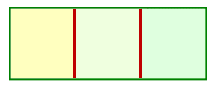Search IntMath
Close

# 3. Permutations (Ordered Arrangements)

An arrangement (or ordering) of a set of objects is called a permutation. (We can also arrange just part of the set of objects.)

In a permutation, the order that we arrange the objects in is important

### Example 1

Consider arranging 3 letters: A, B, C. How many ways can this be done?

The possible permutations are

ABC, ACB,
BAC, BCA,
CAB, CBA.

Hence, there are six distinct arrangements.

Another way of looking at this question is by drawing 3 boxes.Any one of the A, B, C goes into the first box (3 ways to do this), and then the remaining one of the two letters goes into the second box (2 ways to do this), and the last remaining letter goes into the third box (only one way left to do this).

Hence, total no of ways 3 × 2 × 1 = 6

## Reminder - Factorial Notation

Recall from the Factorial section that n factorial (written n!) is defined as:

n! = n × (n − 1) × (n − 2) ... 3 × 2 × 1

Each of the theorems in this section use factorial notation.

## Theorem 1 - Arranging n Objects

In general, n distinct objects can be arranged in n! ways.

### Example 2

In how many ways can 4 different resistors be arranged in series?

Since there are 4 objects, the number of ways is

4! = 24 ways

## Theorem 2 - Number of Permutations

The number of permutations of n distinct objects taken r at a time, denoted by P_r^n, where repetitions are not allowed, is given by

P_r^n =n(n-1)(n-2)...(n-r+1) =(n!)/((n-r)!

### Notes

(1) P_n^n=n! (since 0! = 1)

(2) Some books use the following notation for the number of permutations:

nPr

and others have:

{::}^n P_r

### Example 3

In how many ways can a supermarket manager display 5 brands of cereals in 3 spaces on a shelf?

This is asking for the number of permutations, since we don't want repetitions. The number of ways is:

P_3^5=(5!)/((5-3)!) =(5!)/(2!) =60

### Example 4

How many different number-plates for cars can be made if each number-plate contains four of the digits 0 to 9 followed by a letter A to Z, assuming that

(a) no repetition of digits is allowed?

(b) repetition of digits is allowed?

(a) There are 10 possible digits (0, 1, 2, ..., 9) and we need to take them 4 at a time. There are 26 letters in the alphabet.

With no repetition, we have:

P_4^10xx26=(10!)/((10-4)!)xx26

=(10!)/(6!)xx26

=131040

(b) With repetition, we have simply:

(number of digits from 0000 to 9999) × 26

= 10,000 × 26

= 260,000

## Theorem 3 - Permutations of Different Kinds of Objects

The number of different permutations of n objects of which n1 are of one kind, n2 are of a second kind, ... nk are of a k-th kind is

(n!)/(n_1!xxn_2!xxn_3!xx...xx n_k!

### Example 5

In how many ways can the six letters of the word "mammal" be arranged in a row?

We use Theorem 3.

Since there are three "m"s, two "a"s and one "L" in the word "mammal", we have for the number of ways we can arrange the letters in the word "mammal":

(6!)/(3!xx2!xx1!)=60

## Theorem 4 - Arranging Objects in a Circle

There are (n - 1)! ways to arrange n distinct objects in a circle (where the clockwise and anti-clockwise arrangements are regarded as distinct.)

### Example 6

In how many ways can 5 people be arranged in a circle?

(5 − 1)! = 4! = 24 ways

## Exercises

### Exercise 1

In how many ways can 6 girls and 2 boys be arranged in a row

(a) without restriction?

(b) such that the 2 boys are together?

(c) such that the 2 boys are not together?

(a) This is just 8 people being arranged in a row: 8! = 40,320

(b) Regard the 2 boys as one "unit" and so there are 7 "units" to arrange. This can be done 7! = 5040 ways.

The boys can be arranged in 2! = 2 ways, so the required answer is

7! × 2! = 10,080

(c) There are only 2 possibilities: the boys are together or they are not.

So the number of ways of arranging so that the boys are not together is:

40,320 − 10,080 = 30,240

### Exercise 2

How many numbers greater than 1000 can be formed with the digits 3, 4, 6, 8, 9 if a digit cannot occur more than once in a number?

This is choosing 4 from 5 (any 4 digit number chosen from 3, 4, 6, 8, 9 will be  >1000) plus 5 from 5 (any 5 digit number will be  >1000), where order is important.

So the number of ways we can arrange the given digits so that our resulting number is greater than 1000 such that no digit occurs more than once, is:

P_4^5+P_5^5=(5!)/((5-4)!)+(5!)/((5-5)!)

=(5!)/(1!)+(5!)/(0!)

=240

### Exercise 3

How many different ways can 3 red, 4 yellow and 2 blue bulbs be arranged in a string of Christmas tree lights with 9 sockets?

We use Theorem 3:

(n!)/(n_1!xxn_2!xxn_3!xx...xxn_k!)

So

(9!)/(3!xx4!xx2!)=1260

### Exercise 4

In how many ways can 5 people be arranged in a circle such that two people must sit together?

Regard the 2 people who sit together as one "unit" and the other 3 people as 3 "units". Arrange 4 "units" in a circle:

(4 − 1)! = 3! = 6 ways

Number of permutations of 2 people who sit together: 2! = 2

So

6 × 2 = 12 ways

## Problem SolverThis tool combines the power of mathematical computation engine that excels at solving mathematical formulas with the power of GPT large language models to parse and generate natural language. This creates math problem solver thats more accurate than ChatGPT, more flexible than a calculator, and faster answers than a human tutor. Learn More.